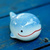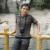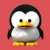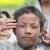## java怎么一个月份分成四周

554330833a 发布于 2016/10/25 21:12

java怎么一个月份分成四周，例如 2.28可以分成 2.1-2.7 2.8-2.14 2.15-2.21 2.22-2.28 ，如果一周有29,30,31天的多出的归到最后的日期段

0```/**
* 获取某年某月的天数
*
* @param year 年
* @param month 月
* @return 总天数
*/
public final static int countDays(int year, int month) {
int count = -1;
switch (month) {
case 1:
case 3:
case 5:
case 7:
case 8:
case 10:
case 12:
count = 31;
break;
case 4:
case 6:
case 9:
case 11:
count = 30;
break;
case 2:
if (year % 4 == 0) {
count = 29;
} else {
count = 28;
}
if ((year % 100 == 0) & (year % 400 != 0)) {
count = 28;
}
}
return count;
}
LocalDateTime ldt = LocalDateTime.now();
int daysOfMonth = countDays(ldt.getYear(), ldt.getMonthValue());00PHP获取月份的天数:
echo date('t', strtotime('2016-02')); //2016年2月的天数
echo date('t'); //当前月份的天数
\$last_week_days = date('t') - 21;0#### 引用来自“BoXuan”的评论

```/**
* 获取某年某月的天数
*
* @param year 年
* @param month 月
* @return 总天数
*/
public final static int countDays(int year, int month) {
int count = -1;
switch (month) {
case 1:
case 3:
case 5:
case 7:
case 8:
case 10:
case 12:
count = 31;
break;
case 4:
case 6:
case 9:
case 11:
count = 30;
break;
case 2:
if (year % 4 == 0) {
count = 29;
} else {
count = 28;
}
if ((year % 100 == 0) & (year % 400 != 0)) {
count = 28;
}
}
return count;
}
LocalDateTime ldt = LocalDateTime.now();
int daysOfMonth = countDays(ldt.getYear(), ldt.getMonthValue());

```public static int getDaysByYearMonth(int year, int month) {

Calendar a = Calendar.getInstance();
a.set(Calendar.YEAR, year);
a.set(Calendar.MONTH, month - 1);
a.set(Calendar.DATE, 1);
a.roll(Calendar.DATE, -1);
int maxDate = a.get(Calendar.DATE);
return maxDate;
}  ```

1.0#### 引用来自“BoXuan”的评论

```/**
* 获取某年某月的天数
*
* @param year 年
* @param month 月
* @return 总天数
*/
public final static int countDays(int year, int month) {
int count = -1;
switch (month) {
case 1:
case 3:
case 5:
case 7:
case 8:
case 10:
case 12:
count = 31;
break;
case 4:
case 6:
case 9:
case 11:
count = 30;
break;
case 2:
if (year % 4 == 0) {
count = 29;
} else {
count = 28;
}
if ((year % 100 == 0) & (year % 400 != 0)) {
count = 28;
}
}
return count;
}
LocalDateTime ldt = LocalDateTime.now();
int daysOfMonth = countDays(ldt.getYear(), ldt.getMonthValue());

```public static int getDaysByYearMonth(int year, int month) {

Calendar a = Calendar.getInstance();
a.set(Calendar.YEAR, year);
a.set(Calendar.MONTH, month - 1);
a.set(Calendar.DATE, 1);
a.roll(Calendar.DATE, -1);
int maxDate = a.get(Calendar.DATE);
return maxDate;
}  ```

1.
```嗯，不过jdk8最新时间日期源码里是下面这么写的：
int dom = 31;
switch (month) {
case 2:
dom = (IsoChronology.INSTANCE.isLeapYear(year) ? 29 : 28);
break;
case 4:
case 6:
case 9:
case 11:
dom = 30;
break;
}